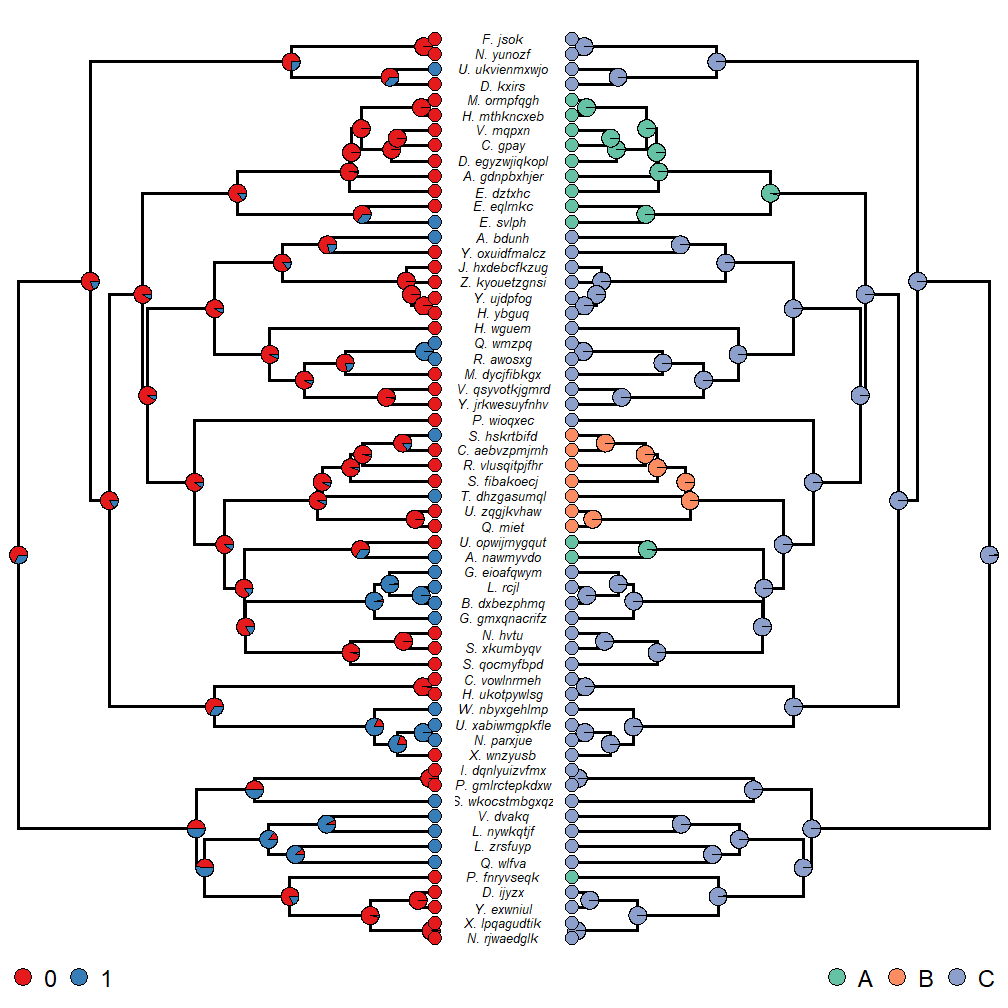## Wednesday, February 21, 2018

### A refresher on plotting facing phylogenies with node labels

An R phylogenetics user today contacted me about plotting two identical facing trees, along with reconstructed ancestral states at internal nodes, but with only one set of tip labels between them.

This is easy enough to do, so let's see how.

First our tree & data are simulated - but with realistic looking tip labels.

``````library(phytools)
library(RColorBrewer)
cw<-untangle(tree,"read.tree") ## make sure tips are in order
layout(matrix(1:3,1,3),widths=c(0.45,0.1,0.45))
plotTree(cw,ftype="off",ylim=c(-1,Ntip(cw)))
obj<-rerootingMethod(cw,x,model="ER")
k<-length(levels(x))
cols<-setNames(brewer.pal(max(c(3,k)),"Set1")[1:k],levels(x))
nodelabels(pie=obj\$marginal.anc,piecol=cols,cex=1)
tiplabels(pie=to.matrix(x,levels(x)),piecol=cols,cex=0.8)
legend(x="bottomleft",legend=levels(x),pch=21,pt.cex=2.5,pt.bg=cols,cex=1.5,
bty="n",horiz=T)
ylim<-get("last_plot.phylo",envir=.PlotPhyloEnv)\$y.lim
plot.new(); plot.window(xlim=c(-0.1,0.1),ylim=ylim)
text(rep(0,Ntip(cw)),1:Ntip(cw),gsub("_"," ",cw\$tip.label),cex=0.8,
font=3)
plotTree(cw,ftype="off",ylim=c(-1,Ntip(cw)),direction="leftwards")
obj<-rerootingMethod(cw,y,model="ER")
k<-length(levels(y))
cols<-setNames(brewer.pal(max(c(3,k)),"Set2")[1:k],levels(y))
nodelabels(pie=obj\$marginal.anc,piecol=cols,cex=1)
tiplabels(pie=to.matrix(y,levels(y)),piecol=cols,cex=0.8)
legend(x="bottomright",legend=levels(y),pch=21,pt.cex=2.5,pt.bg=cols,cex=1.5,
bty="n",horiz=T)
``````Of course, some of the plotting parameters - such as the font size, line width, and the sizes of the plotted pies - will need to be adjusted depending on the size of our tree; but hopefully this is a good starting point!

FYI, here's how the tree & data were simulated:

``````N<-60
tips<-paste(sample(LETTERS,N,replace=T),"._",
replicate(60,paste(sample(letters,sample(4:12,1)),
collapse="")),sep="")
tree<-pbtree(n=N,tip.label=tips,scale=1)
Q1<-matrix(c(-1,1,1,-1),2,2,dimnames=list(0:1,0:1))
x<-sim.Mk(tree,Q1)
Q2<-matrix(c(-0.4,0.2,0.2,0.2,-0.4,0.2,0.2,0.2,-0.4),3,3,
dimnames=list(LETTERS[1:3],LETTERS[1:3]))
y<-sim.Mk(tree,Q2)
``````